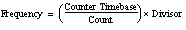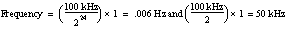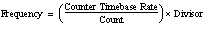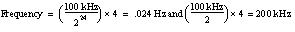# Large-Range Two Counter Measurement Method

If you measure the digital frequency or the period of a counter signal, you can use this two-counter method to measure signals with large ranges. This method is useful when you have a widely varying signal to measure and would like increased accuracy throughout the entire range. Refer to the quantization error section for more information on increasing measurement accuracy with the large-range measurement method. You can also use this method to measure signal frequencies that are faster than your counter timebase rate as long as the input signal does not exceed the maximum input frequency supported by the counter.

To perform measurements using this method in NI-DAQmx, a paired counter is used to divide the input signal by a value specified using the Divisor attribute/property. However, you need to be careful the Divisor you choose does not cause the counter to roll over. This divisor has the effect of shifting the measurable frequency range upward. The Divisor scales the measured period and returns data according to the following equations:

Period = Measured Period / Divisor

Frequency = Divisor × Measured Period

For example, if you use a 24-bit counter and the Counter Timebase Rate is 100 kHZ, the measurable frequency range is approximately 0.006 Hz to 50 kHz becauseHowever, with a divisor of 4, the measurable frequency range is 0.024 Hz to 200 kHz becauseTo determine if you should use the large-range measurement method, refer to the quantization error tables. If the quantization error listed for the one-counter method in that section is too high, use the large-range measurement method instead.